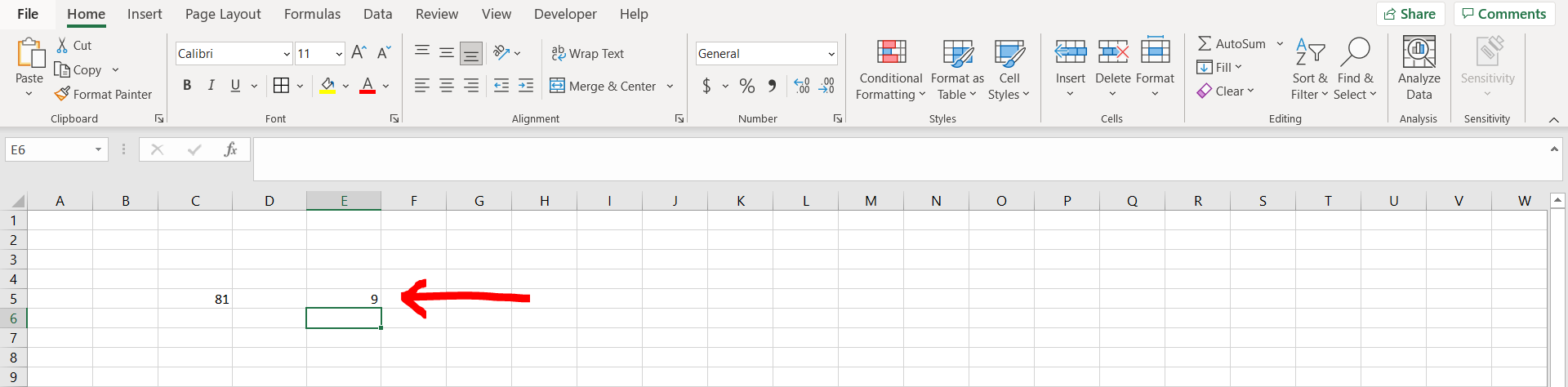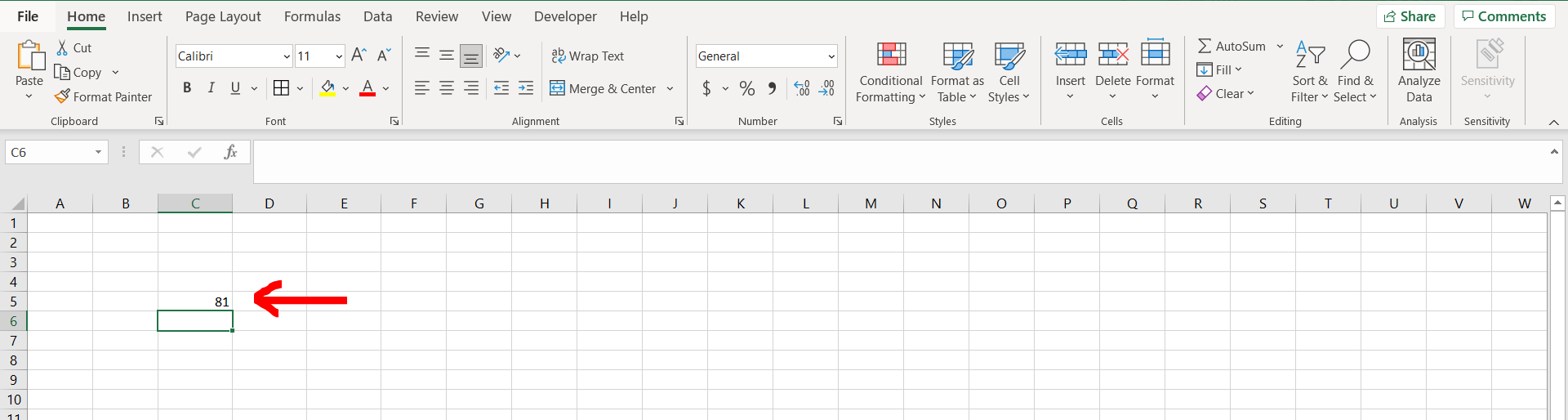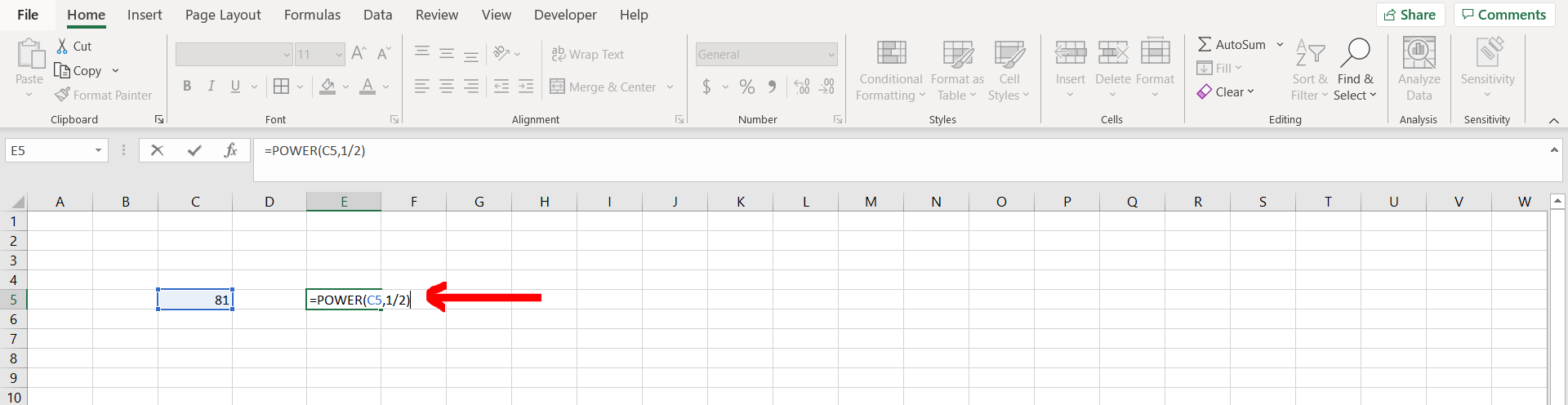# How to do square root on ExcelExcel offers a very interesting way to do square root. We can cater to this problem statement by using the “=power” formula. We can perform the below mentioned way to do square root in excel:

### Step 1 – Excel workbook with a square– Open the desired Excel workbook containing a square which needs to be square rooted

### Step 2 – Using the power formula– Now enter “=POWER(“ and then give reference to the cell which needs to be cube rooted followed by a comma, and then type “1/2”, and hit Enter.

### Step 3 – Square root obtained– We can see that the square root of the number has been obtained